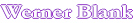Programs for Polymer and Coating Formulation Design

Home
Polymer Synthesis
Acrylic and Vinyl Polymer, Tg of copolymer with as many six monomers. As input the weight amount of co-monomer is required. Output is in degree Kelvin and Centigrade. More than 120 monomers can be selected. If you are interested in a monomer not listed, let me know and I can modify the program Fox Equation
Tg, solubility parameter, viscosity and density of polymers and oligomers can be calculated using an  using cohesive energy approach according to Feddors.  Cohesive energy
Tg, solubility parameter, cohesive energy, specific gravity and viscosity from polymer segments can be calculated using the method according to Krevelen. Krevelen
Polyester synthesis from polyols, carboxylic acids and lactones. Weight charges, molecular weight and functionality of the resulting polyol are calculated as a function of conversion and loss of polyol Polyester
Polymer Properties
Crosslink density calculation from swelling measurements using the Flory-Rehner equation. More than 400 solvents and reactants can be selected for the swelling calculation. Dichloromethane (methylene chloride) is a solvent of choice for this measurement and give very fast swell results. Some higher boiling and more viscous solvents might need extensive times to reach swelling equilibrium. Swelling
Molecular weight from osmotic pressure measurements. Osmotic pressure of polymer solutions can be used to calculated the number average molecular weight of polymers. Osmotic pressure
Viscosity-Solids relationship of a polymer solution. The viscosity of a polymer solution can be calculated from two data points using the assumption that the glass transition temperature (Tg) of a polymer decreases linear with the weight fraction of added solvent.  Viscosity-solids
Viscosity of a polymer melt or solution over a temperature range. The William Landel Ferry equation (WLF) permits the calculation of the viscosity of a polymer melt or solution more accurate then the Arrhenius equation. Viscosity-temperature
Viscosity of a blend of up to four polymer melts or solutions. This program utilizes the William Landel Ferry equation (WLF) in conjunction with the Fox equation to calculate the viscosity of polymer blends at different temperatures. It is assumed that no specific interaction between the polymers is taking place and that the polymers are compatible. As input polymer viscosity, temperature and non-volatile is required. Viscosity-blends
Viscosity of a blend of polymer solutions. A combination of the WLF, Fox equation is used to calculate the viscosity of blends of two polymer solutions. As input two viscosity-solids data points of each polymer solution is required. The program permits to calculate the viscosity at any solids and temperature and mix ratio of the two polymer solutions.  Viscosity polymer solutions
Formulation
Dispersant demand of spherical particles. Calculates the demand of dispersants as a function of particle diameter and thickness of the absorption layer. Dispersant spherical
Dispersant demand of elongated particle (prolate ellipsoid). Calculates the dispersant demand of an elongated particle. Dispersant elongated
Volume & Surface Area of Particles and Number of Molecules. This program calculates the volume, weight and number of molecules in a spherical particle and the surface layer. Volume surface
Volume & Surface Area of Particles and Number of Molecules. This program calculates the volume, weight and number of molecules in a spherical particle and the surface layer, both the particle and the shell can be swollen with a solvent. Volume swollen
Sedimentation (settling) of particles. The Stokes equation permits the estimation of settling speed of spherical particles at a viscous liquid at low Reynolds numbers. Sedimentation
Sagging of a liquid film as a function of film thickness and viscosity. Coating films on vertical surfaces sag as a function of viscosity, film thickness and gravity. Sagging
Flow and leveling of coatings. Most application processes result in coating which have a wavy surface and require leveling to achieve the desired appearance. The Orchard equation permits the calculation of leveling of a coating film as a function of striation, film thickness, viscosity and surface tension. Leveling
Viscosity of pigmented polymers. The Mooney equation permits the calculation of the viscosity of pigmented polymer solutions or polymer melts as a function of the volume fraction of pigment. The viscosity of flocculated and un-flocculated systems can be calculated the effect of an adsorbed resin layer can also be  considered. As input into the equation the viscosity characteristics of as many as four polymer solutions or melts can be used. The constants used are for spherical pigment particles and can be adjusted for non-spherical pigments. Viscosity pigmented polymer
Viscosity of pigmented polymers. This program is an expansion of above Mooney equation which permits the selection of up to 4 different pigments. Because of the potential for increased packing of spheres the program will not work with pigments with large difference in particle size. Viscosity pigmented polymer up to 4 pigments
Solvent properties
Solvent properties. Find the CAS #, boiling point, molecular weight, density, refractive index, vapor pressure and flash point of more than 400 solvents. Solvents
Solvent selection. Select a solvent based on desired boiling point and increments in boiling point Solvent selection
Solvent selection. Select a solvent based on desired boiling point and solubility parameter Boiling Point, Solubility
Vapor pressure of a solvent. Calculate the vapor pressure and boiling point of a solvent based on the Antoine equation which is derived from the Claussius-Clapeyron equation Vapor pressure
Vapor pressure of a blend up to four solvents.  Using the Antoine equation and Raoult's law this program calculates the vapor pressure of as many of four solvents at different temperatures. It also determines the composition of the vapor phase. The accuracy of the method depends how "ideal" the solvent properties are.  Vapor pressure solvents
 Last edited on: February 08, 2013 Copyright®, Design, Layout and Technical Content by: